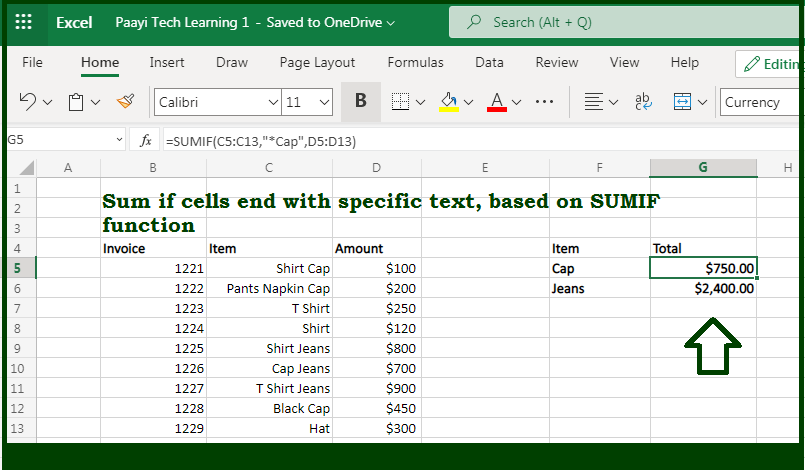# Learn How to SUM If Ends With in Microsoft Excel

Written by | 0 Comments | 361 Views

In this article, you will learn how to SUM various things in Microsoft Excel using a single/combination(s) of functions. You will also know how to SUM If Ends With and see the generic formula.

SUM If Ends With Specific Text in Microsoft Excel

The main purpose of this formula is to sum if cells end with specific text. Here we will learn how to sum if the date is ending with some specific text in the workbook in Microsoft Excel. That implies, with the help of a formula based on the SUMIF function you can able to sum if cells end with specific text. So, with the help of this formula, you can able to sum if the date is ending with some specific text in the workbook in Microsoft Excel.

General Formula to SUM If Ends With

=SUMIF(range,"*text",sum_range)

The Explanation for the SUM If Ends WithSo we know that with the help of the given formula above you can able to sum if cells end with specific text. Here we will learn how to sum if the date is ending with some specific text in the workbook in Microsoft Excel. As we know that the SUMIF function supports  logical operators efficiently, which are -  "=",">",">=", etc. So you can able to use these operators as per your criteria. Moreover, the SUMIF formula is dependent upon wildcards. An asterisk (*) used as a wildcard means "one or more characters", while a question mark (?) means "any one character". With this feature, you can able to construct criteria such as "begins with", "ends with", "contains 3 characters", and so on. So, with the help of this formula, you can able to sum if the date is ending with some specific text in the workbook in Microsoft Excel.